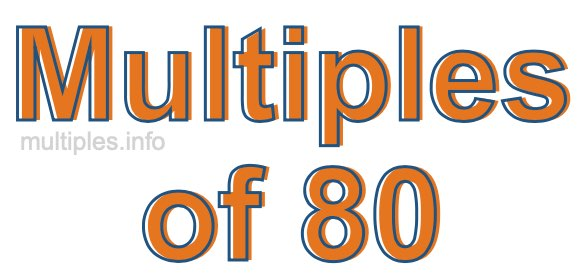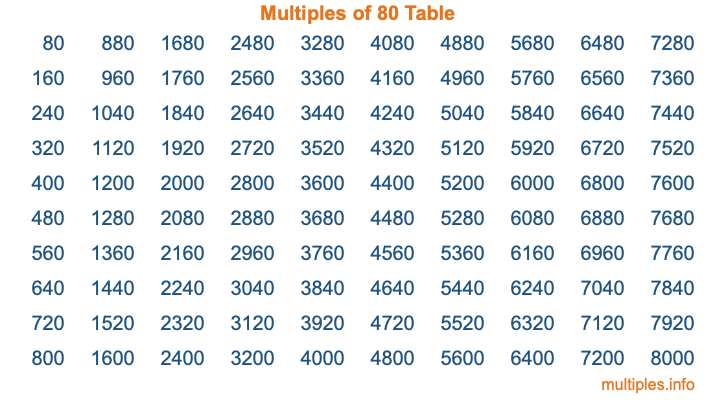Multiples of 80Welcome to the Multiples of 80 page. Here we will first teach you everything you will ever need to know about the multiples of 80, and then give you a study guide summary of everything we taught you to make sure you remember it all. Use this page to look up facts and learn information about the multiples of 80. This page will make you a multiples of eighty expert!

Definition of Multiples of 80
Multiples of 80 are all the numbers that when divided by 80 equal an integer. Each of the multiples of 80 are called a multiple. A multiple of 80 is created by multiplying 80 by an integer.

Therefore, to create a list of multiples of 80, you start with 1 multiplied by 80, then 2 multiplied by 80, then 3 multiplied by 80, and so on for as long as you want. Thus, the list of the first five multiples of 80 is 80, 160, 240, 320, and 400. To see a larger list of multiples of 80, see the printable image of Multiples of 80 further down on this page. We also have a category where you can choose any nth multiple of 80.

Multiples of 80 Checker
The Multiples of 80 Checker below checks to see if any number of your choice is a multiple of 80. In other words, it checks to see if there is any number (integer) that when multiplied by 80 will equal your number. To do that, we divide your number by 80. If the the quotient is an integer, then your number is a multiple of 80.

Is  a multiple of 80?

Least Common Multiple of 80 and ...
A Least Common Multiple (LCM) is the lowest multiple that two or more numbers have in common. This is also called the smallest common multiple or lowest common multiple and is useful to know when you are adding our subtracting fractions. Enter one or more numbers below (80 is already entered) to find the LCM.

Check out our LCM Calculator if you need more details about the Least Common Multiple or if you need the LCM for different numbers for adding and subtraction fractions.

nth Multiple of 80
As we stated above, 80 is the first multiple of 80, 160 is the second multiple of 80, 240 is the third multiple of 80, and so on. Enter a number below to find the nth multiple of 80.

th multiple of 80

Multiples of 80 vs Factors of 80
80 is a multiple of 80 and a factor of 80, but that is where the similarities end. All postive multiples of 80 are 80 or greater than 80. All positive factors of 80 are 80 or less than 80.

Below is the beginning list of multiples of 80 and the factors of 80 so you can compare:

Multiples of 80: 80, 160, 240, 320, 400, etc.

Factors of 80: 1, 2, 4, 5, 8, 10, 16, 20, 40, 80

As you can see, the multiples of 80 are all the numbers that you can divide by 80 to get a whole number. The factors of 80, on the other hand, are all the whole numbers that you can multiply by another whole number to get 80.

It's also interesting to note that if a number (x) is a factor of 80, then 80 will also be a multiple of that number (x).

Multiples of 80 vs Divisors of 80
The divisors of 80 are all the integers that 80 can be divided by evenly. Below is a list of the divisors of 80.

Divisors of 80: 1, 2, 4, 5, 8, 10, 16, 20, 40, 80

The interesting thing to note here is that if you take any multiple of 80 and divide it by a divisor of 80, you will see that the quotient is an integer.

Multiples of 80 Table
Below is an image of the first 100 multiples of 80 in a table. The table is in chronological order, column by column. The first column has the first ten multiples of 80, the second column has the next ten multiples of 80, and so on.The Multiples of 80 Table is also referred to as the 80 Times Table or Times Table of 80. You are welcome to print out our table for your studies.

Negative Multiples of 80
Although not often discussed or needed in math, it is worth mentioning that you can make a list of negative multiples of 80 by multiplying 80 by -1, then by -2, then by -3, and so on, to get the following list of negative multiples of 80:

-80, -160, -240, -320, -400, etc.

Multiples of 80 Summary
Below is a summary of important Multiples of 80 facts that we have discussed on this page. To retain the knowledge on this page, we recommend that you read through the summary and explain to yourself or a study partner why they hold true.

There are an infinite number of multiples of 80.

A multiple of 80 divided by 80 will equal a whole number.

80 divided by a factor of 80 equals a divisor of 80.

The nth multiple of 80 is n times 80.

The largest factor of 80 is equal to the first positive multiple of 80.

80 is a multiple of every factor of 80.

80 is a multiple of 80.

A multiple of 80 divided by a divisor of 80 equals an integer.

80 divided by a divisor of 80 equals a factor of 80.

Any integer times 80 will equal a multiple of 80.

Multiples of a Number
Here you can get the multiples of another number, all with the same attention to detail as we did for multiples of 80 on this page.

Multiples of
Multiples of 81
Did you find our page about multiples of eighty educational? Do you want more knowledge? Check out the multiples of the next number on our list!

Copyright  |   Privacy Policy  |   Disclaimer  |   Contact